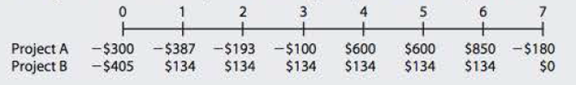Chapter 11, Problem 17P### Fundamentals of Financial Manageme...

9th Edition
Eugene F. Brigham + 1 other
ISBN: 9781305635937

#### Solutions

Chapter
Section### Fundamentals of Financial Manageme...

9th Edition
Eugene F. Brigham + 1 other
ISBN: 9781305635937
Textbook Problem
38 views

# CAPITAL BUDGETING CRITERIA A company has an 11% WACC and is considering two mutually exclusive investments (that cannot be repeated) with the following cash flows:a. What is each project’s NPV? b. What is cach project’s IRR? c. What is each project’s M1RR? (Hint: Consider Period 7 as the end of Project B’s life.) d. From your answers to parts a, b, and c, which project would be selected? If the WACC was 18%, which project would be selected? e. Construct NPV profiles for Projects A and B. f. Calculate the crossover rate where the two projects’ NPVs are equal. g. What is each project’s MIRR at a WACC of 18%?

a.

Summary Introduction

To calculate: The NPV of the Project A and Project B.

Introduction:

Mutually Exclusive Projects:

It refers to the group of projects in which, if one project is accepted, it will automatically imply the rejection of the rest. It refers to those projects for which investment cannot be made together.

Net Present Value (NPV):

It is a method under capital budgeting, which includes the calculation of net present value of the project in which company is investing. The calculation is done by calculating the difference between the value of cash inflow and value of cash outflow after taking into consideration the discounted rate.

Explanation

The calculation of NPV for Project A on spreadsheet,

Table (1)

The NPV for Project A is -\$148.80...

b.

Summary Introduction

To calculate: The IRR for Project A and Project B.

Introduction:

Internal Rate of Return (IRR):

It refers to the rate of return that is computed by the company to make a decision on the selection of a project for investment. This rate provides the basis for selection of projects with lower cost of capital and rejection of project with higher cost of capital.

c.

Summary Introduction

To calculate: MIRR for the project A and project B.

Introduction:

Modified Internal Rate of Return (MIRR):

It refers to the rate of return that is computed by the company to make a decision of the selection and ranking of a project for investment. This is a modified version of IRR with reinvestment of cash flows at the cost of capital.

d.

Summary Introduction

To explain:  Whether the Project A or Project B should be selected on the basis of calculation made in part a, b, c and when the WACC is 18%.

e.

Summary Introduction

To construct: NPV profiles for Project A and Project B.

f.

Summary Introduction

To calculate: The crossover rate of the two projects.

Introduction:

Crossover Rate:

It refers to the discounted rate at which the NPV of the two projects becomes equal. It is a cost of capital of the project.

g.

Summary Introduction

To calculate: The MIRR for Project A and Project B at 18% WACC.

### Still sussing out bartleby?

Check out a sample textbook solution.

See a sample solution

#### The Solution to Your Study Problems

Bartleby provides explanations to thousands of textbook problems written by our experts, many with advanced degrees!

Get Started